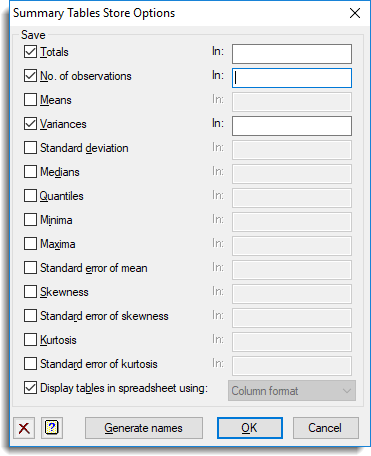1. Home
2. Summary Tables Store Options

# Summary Tables Store Options

Use this to save summary information for grouped data. These results are saved when you click Run in the main Summary Tables dialog.

1. After selecting the appropriate boxes, type names for the data structures into the corresponding In: fields.
Alternatively, click Generate names to auto-fill the fields with names.

The table below indicates the type of structures formed for each item.## Save

 Totals Table The totals for each group. No. of observations Table The number of observations in each group. Means Table The arithmetic mean for each group. Variances Table The variance of the values for each group. Standard deviation Table The standard deviation of the values for each group. Medians Table The median value in each group. Quantiles Table or Pointer The quantiles for each group. If a list of two or more quantiles is specified, the results will be stored in a pointer which points to individual tables for each quantile. Minima Table The minimum value in each group. Maxima Table The maximum value in each group. Standard error of mean Table The standard error of mean of the values for each group. Skewness Table The skewness of the values for each group. Standard error of skewness Table The standard error of skewness of the values for each group. Kurtosis Table The kurtosis of the values for each group. Standard error of kurtosis Table The standard error of kurtosis of the values for each group.

## Display tables in spreadsheet using

Select this to display the results in a new spreadsheet window. The format of the spreadsheet can be controlled by the choices in the dropdown list.

 Page format Each table is displayed on a single page in a separate spreadsheet. The last specified classifying factor indexes the columns in the spreadsheet. Column format The tables occupy a single column but multiple tables can be put in a single spreadsheet. This is the default for a table with a single classifying factor. Tabbed format Each table is displayed on a single page in a tabbed-table spreadsheet. The separate tables appear as tabs in the spreadsheet and manipulations on one table are propagated to all other tables in the book. If there is only a single table with three or more classifying factors being saved, then the table will be displayed as a tabbed-table with the first classifying factor’s groups indexing the tabs in the book.